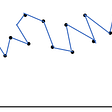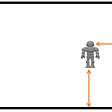# Activation functions for Neural Networks

When building a neural network, we need to choose an activation function for the layers of the neural network. The main purpose of an activation function is to introduce non-linearity into the output of a neuron. Without using activation functions, the layers of a Neural Network will simply linearly transform the inputs, which will never give the desired results, except maybe in some linear regression problems.

Here I am going to discuss the four most common activation functions used in Deep Learning, along with their relative advantages and disadvantages and their derivatives, which in turn is useful for calculating gradients.

Sigmoid Function

The sigmoid activation function is perhaps the first one everyone learns about when foraying into the field of machine learning or deep learning. The function is given by

`σ(x) = 1/(1+exp(-x))`

When plotted the sigmoid function looks like this

We can see that the value of σ(x) ranges from 0 to 1. This is especially useful in binary classification problems where it flattens any input to outputs between 0 and 1.

One major disadvantage with the sigmoid function is that the gradients or the slope at values of x greater than 4 or less than -4 are almost 0. This leads to poor learning when we use a gradient descent algorithm to update the value of our weights and intercepts.

Also, it has been seen that the tanh activation function almost always performs better in a neural network than the sigmoid function. So sigmoid activation function is rarely used in neural networks, except when we have a binary classification problem, we use it in the final layer.

The derivative of the sigmoid function is given by

`σ(x) = σ(x)(1-σ(x))                                              (1)`

Tanh activation function

Tanh activation function is given by

One reason tanh is preferred over sigmoid function for neural networks is that it is centred around 0, which means the outputs will also be centred around 0, leading to faster convergence, compared to when the outputs are centred around 0.5, as seen in the case of sigmoid.

Tanh activation function also suffers from the issue of vanishing gradients as the values of x increase or decrease above +4 or below -4.

The derivative of tanh function is given by

`tanh'(x) = 1 - tanh(x)*tanh(x)`

ReLU activation function

ReLU or Rectified Linear Unit activation function is the one that is most widely used nowadays for neural networks. The main advantage of ReLU over the other 2 activation functions mentioned before is, that the slope is always 1 for values of x > 0.

ReLU is given by

ReLU has been observed to perform better in most cases than tanh and sigmoid and is thereby widely used today. One disadvantage with ReLU is that the slope for negative input is zero, which means for activations in that region, the weights are not updated during backpropagation. This can create dead neurons that never get activated.

The derivative of ReLU is given by

Leaky ReLU activation function

Leaky ReLU activation function is a slight modification of the ReLU activation function as can be seen below

In the case of Leaky ReLU, the gradient for values less than 0, is not 0, and this helps increase the range of the ReLU function.

The derivative for Leaky ReLU is given by

To conclude, ReLU activation functions are the most widely used activations functions today in Deep Learning, along with its many variations, one of which we discussed above. Tanh and sigmoid activation functions can also be used in some cases, but ReLU has been observed to almost always perform better.

The ideas are from DeepLearning.AI which I have tried to reproduce in my own words.

--

--

--

## More from Prakhar S

Data Scientist, Kode Tiger

Love podcasts or audiobooks? Learn on the go with our new app.

## Probability Learning II: How Bayes’ Theorem is applied in Machine Learning## The problem of Overfitting in Regression and how to avoid it?## AUTOXGBOOST + Optuna## Using LSTM To Navigate Robots## Brain Tumor MRI segmentation using Deep Learning.## ML Day 1 — Introduction to ML!!!## Research Papers based on developments in Human Activity Recognition part1 (Computer Vision)## The NLP Cypher | 03.28.21## Prakhar S

Data Scientist, Kode Tiger

## Naive Bayes Classifier Part-1 (mathematical approach)## Gaussian Processes Demystified## A Beginner’s Guide to Activation Functions## Let’s Learn: Neural Nets #1